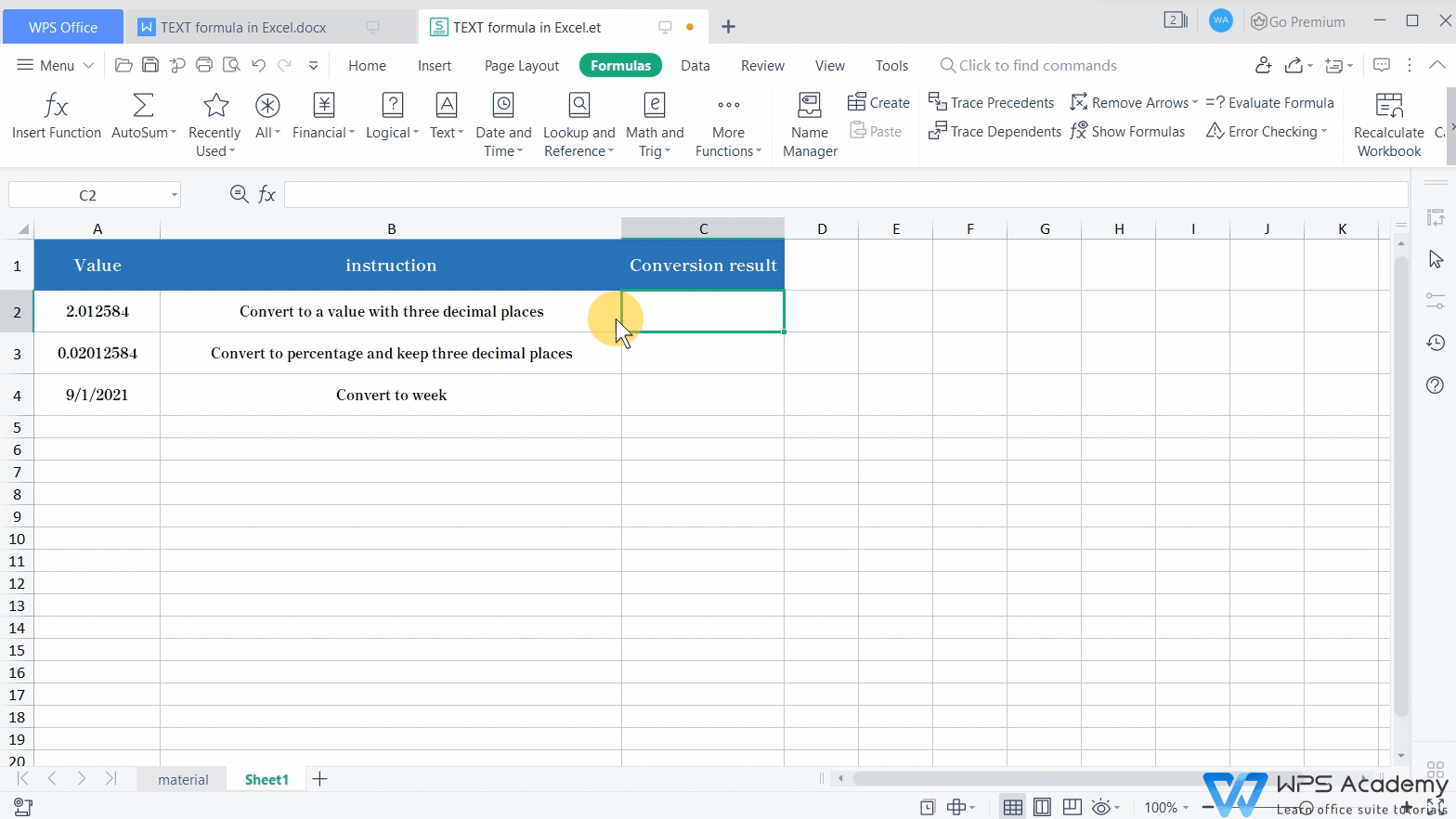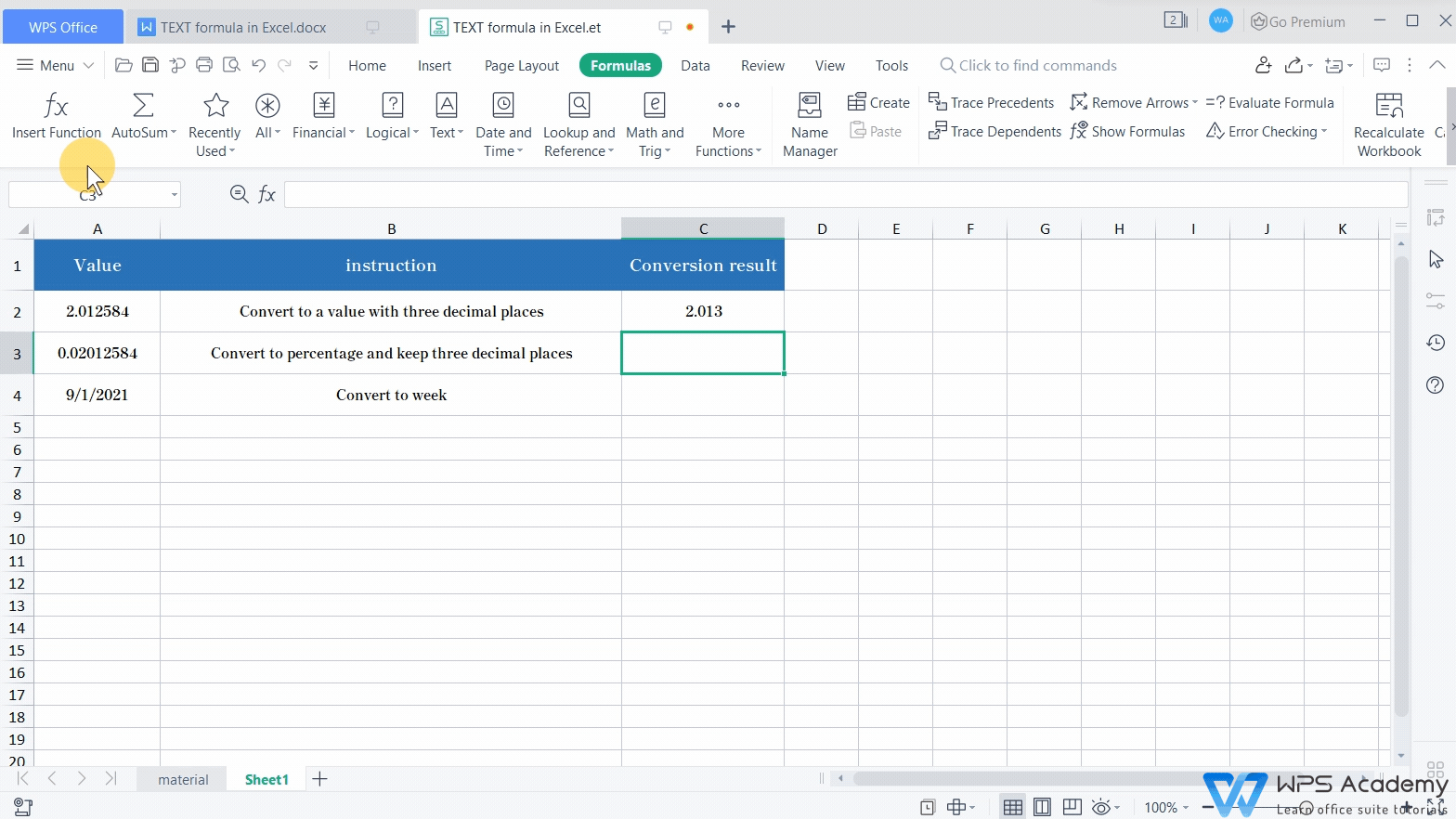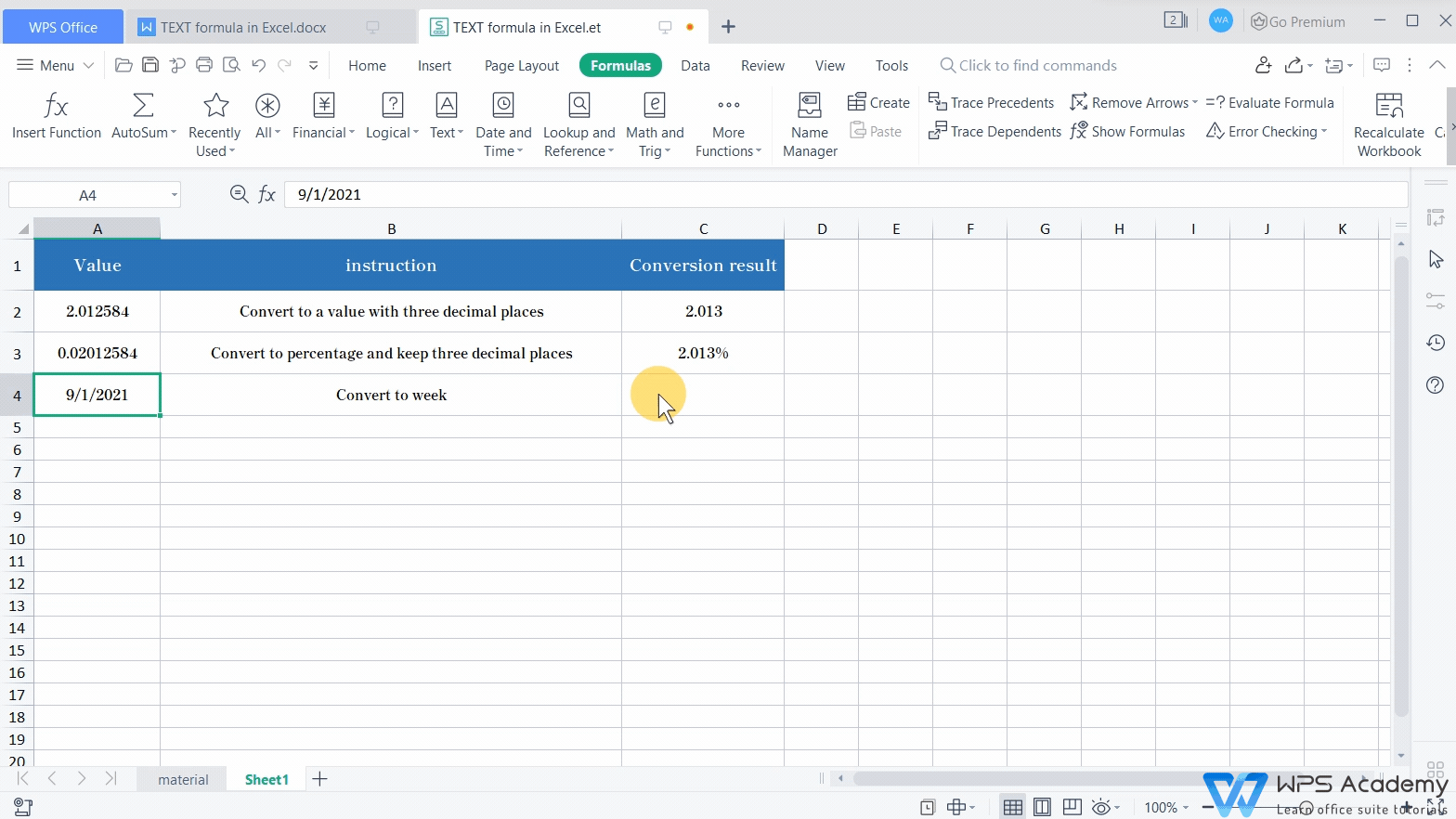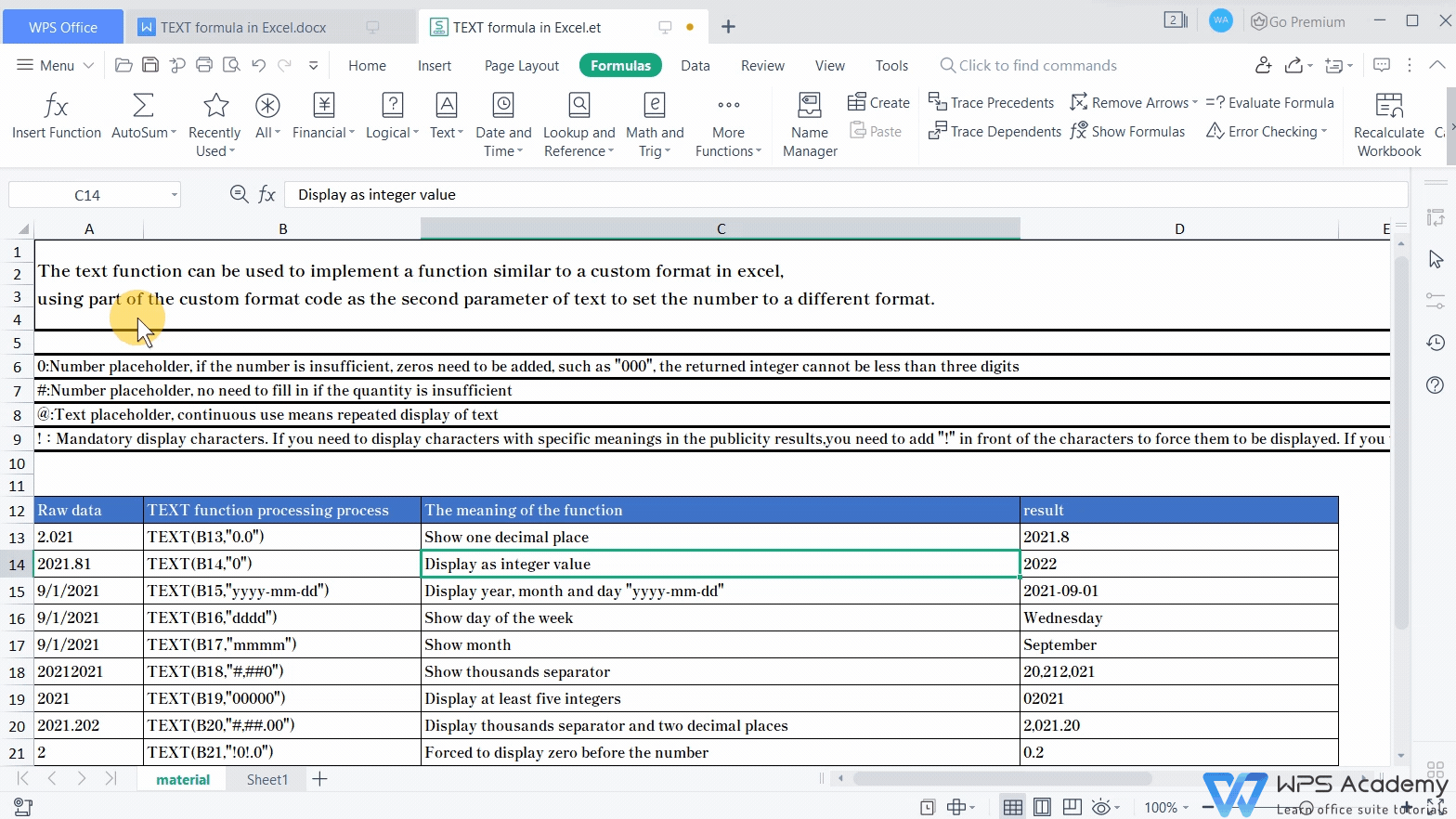# TEXT formula in WPS Spreadsheet

Uploaded time: August 27, 2021 Difficulty: Beginner# TEXT formula in WPS Spreadsheet

The TEXT formula in Excel is commonly used for the conversion of number-to-text in a certain format, and it could be also used in Microsoft Office Excel and Openoffice.

Normally, the TEXT formula is used to customize format cells in terms of actual needs. To realize it, we need to adjust the second parameter of the formula, which stands for format texts to be set. In this example, the number 2.012584, which equips with six decimal places, entered cell A2. How can we convert it into a value with only three decimal places?

1. Select cell C2.

2. Click Insert Function in the Formula tab, and choose the TEXT formula from the popup dialog.

This step can insert the TEXT formula into cell C2. Here Value corresponds to the number in A2, while the Format_text means the expected format for the target value.In this example, select cell A1 when the Value box is in editing status. Then, enter 0.000 as the target format in the Format_text box, which means the target value will present as a number with three decimal places. Note that quotation marks are needed if we want the target value to present as pure text contents. Finally, click OK to get the target value 2.013.Here is another case. If we want to convert the value of cell A3 into a percentage with three decimal places, the same format as 0.000%, the TEXT formula can also be helpful.

1. Click the Insert function button, and insert the TEXT formula.

2. Fill the Value box by selecting cell A3.

3. Enter 0.000% in the Format_text input box, and click OK to get the result.Similarly, we can run the TEXT formula to indicate days of the week for the exact date. For instance, the result indicates that it is Wednesday on September 1st, 2021. To realize it, click the Insert function button, and insert the TEXT formula. Then, enter the following parameter into the corresponding input box: A4 in the Value input box and dddd in the Format_text box. Here dddd stands for days of the week. After these, click OK to run the formula.Here comes the end of the introduction to the TEXT formula. A list of functions of the TEXT formula is presented on the screen. You can also download relevant materials for practice from the link added in the description box of this video.